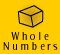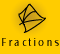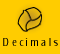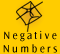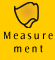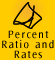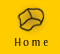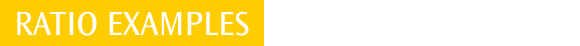| Expressing Relationships as Ratios | Sharing Ratios |
| Map Scales | Comparing value for money | Quick quiz |

Expressing Relationships as Ratios

The following examples shows how ratios can be used to express many different relationships between a number of quantities or people.

 Example 1: A take away food store finds that they sell burgers in the following ratios Super Burgers : Basic Burgers : Vegie Burgers = 25 : 10 : 2 What is the ratio in simplest terms for a) Super : Basic b) Basic : Vegie c) Basic : Super d) Vegie : Super

 Working Out a) Super : Basic = 25 : 10. I could choose to simplify this ratio further, to 5:2 b) Basic : Vegie = 10 : 2. The equivalent ratio in simplest terms would be 5 : 1 c) Basic : Super = 10 : 25. I could then simplify this ratio to 2:5 (Note that we are making a similar comparison to (a) but that the order of the ratio has changed.) d) Vegie : Super = 25 : 2. I could choose to re-express this ratio as 12.5 : 1 but most people prefer both to be whole numbers.

Example 2: A childcare centre requires a minimum caregiver to child ratio of 1 : 5. Work out how many caregivers are required, or children able to attend, for each day of the week.

a) Monday: How many caregivers are needed if 25 children attend?
b) Tuesday: If only 4 caregivers are available how many children can attend?
c) Wednesday: How many caregivers are needed if 33 children attend?
d) Thursday: How many caregivers are needed if 26 children attend?
e) Friday: If only 5 caregivers are available, can 30 children attend?

 Working Out Thinking a) 25/5 = 5 5 caregivers are required I need a 1:5 ratio of caregiver to child, this means I need 1 caregiver for every 5 children. To find out how many caregivers I need I can divide the number of children by 5. b) 4 caregivers x 5 children/caregiver 20 children can attend Only 4 caregivers are available. The maximum number of children each caregiver can look after is 5, therefore 5 x 4 children can attend. c) 33/5 = 6 and 3 left over 7 caregivers are required 33 children divided by 5 gives 6, with 3 children left over. This means I need an extra caregiver for the 3 extra children. d) 26/5 = 5 and 1 left over 6 caregivers are required 26 children divided by 5 gives 5, with 1 child left over. This means I need an extra caregiver for the extra child. e) 5 caregivers x 5 children/caregiver 25 children can attend (30 children cannot attend) Only 5 caregivers are available. The maximum number of children each caregiver can look after is 5, therefore 5 x 5 children can attend.

Example 3: Local primary school students were surveying who was borrowing books at the local library. Over a 1 week period it was found that 200 people borrowed books: 110 people were women, 50 were men and the rest were children. Express the following relationships as ratios.

 a) the number of women (borrowers) to the number of children (borrowers) b) the number of children to the number of men c) the number of men to total number of borrowers d) the number of men to the number of women e) the number of women to the number of men f) the number of women to total number of borrowers

 Thinking a) There are 110 women. There are 200 - 110 - 50 = 40 children. The ratio of the number of women to children is 110:40. We can simplify this ratio to 11:4 b) There are 50 men and 40 children. The ratio of the number of men to the number of children is 50:40. We can simplify this ratio to 5:4. c) There are 50 men. There are 200 people in total. The ratio of the number of men to the number of total people is 50 : 200. This ratio can be simplified to 1:4. d) There are 110 women and 50 men. The ratio of women to men is 110 : 50. This ratio can be simplified to 11:5. e) There are 50 men and 110 women. The ratio of men to women 50 : 110. We can simplify this ratio to 5:11. f) There are 110 women. There are 200 people in total. The ratio of the number of women to the number of total people is 110 : 200. We can simplify this ratio to 11:20.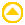Sharing Ratios

Example 4: movie - sharing ratios. A prize of \$450 needs to shared in the ratio of 1:2

Example 5: movie - sharing ratios.
Three brothers pool their money for a \$100 raffle ticket for a car. Jarrod puts in \$20, Jeremy puts in \$20 and Gerald puts in \$60. The prize, a four wheel drive vehicle, is worth \$60 000. If they win the car, what is the dollar value of each brothers share?

The movie below shows a another, shorter method to calculate the answerMap Scales

A very common use of ratios is for map scales. A street directory might say it uses a scale where 1 cm represents 100 metres. To write this as a ratio both quantities must be in the same units.

1 cm represents 100 metres so we convert 100 metres into centimetres,

100 m x 100 = 10 000 centimetres
1 cm represents 10 000 centimetres

We can now write the ratio as 1 : 10 000. This ratio is called a scale factor. The scale factor is what we generally look for when we are trying to work out what distance a portion of a map represents.

A scale factor of 1 : 10 000 tells us that 1 cm on the map represents 10 000 cm on the ground, 1 inch on the map represents 10 000 inches on the ground and 1 finger width on the map represents 10 000 finger widths on the ground.

Example 6: movie - map scales.
On an architect's drawing 1 cm represents 0.5 of a metre, what is the scale factor?

What would 1 inch on the drawing represent?

Example 7: movie - map scales.
On a map in which 1 cm represents 5 km, calculate the scale factor (express the scale in ratio form).

What is the real distance measured by 9 cm on the map?

The speaker makes a mistake towards the end of this movie, can you pick it up?
'9 cm on the map equals ..... on the ground?'

If the actual distance is 35 km, what distance is this on the map?Comparing value for money

A common application of ratios is comparing prices of similar products but different quantities at the supermarket.

Example 8: Which of these two bottles of tomato sauce is most economically priced?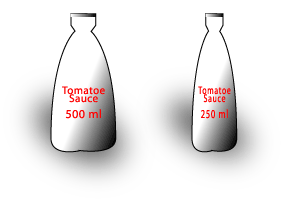500 ml bottle is \$2.74 250 ml bottle is \$1.55

Without using a calculator we can estimate which bottle of sauce is the best buy. The larger bottle is twice the mass of the smaller bottle, so the mass ratio is 2:1. The price of the larger bottle is less than twice the price of the smaller bottle, so the larger bottle is better value for money.

Example: Which of these bags of sugar is most economically priced?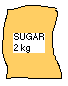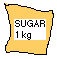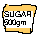2kg bag of sugar is \$2.75 1 kg bag of sugar is \$1.25 500 g bag of sugar is 85 cents

 Thinking The mass ratio of the bags of sugar is 2 : 1 : 1/2 The price ratio of the bags of sugar is 2.75 : 1.25 : 0.85 We can see that the 500g is not a bargain since the price per 1/2 kg is much more than half the price for the 1 kg bag. The price for the 2 kg bag is more than double the price for the 1 kg bag so it is not the cheapest either. Therefore the 1 kg bag of sugar is the best value.

Quick quiz

1. A cafe finds that cappuccinos are sold in the following ratios 3 : 20 : 8 = baby size: regular size: mug size. What is the ratio in simplest terms for,
a) baby to mug
b) baby to regular
c) mug to regular
d) mug to baby

2. The fish pond at the back of the garden has 2 turtles, 7 orange fish, 3 mottly coloured fish and 12 tiny black fish. What the are following ratios?
a) turtles : fish
b) orange fish : black fish
c) turtles : black fish
d) mottly fish : total number of fish
e) mottly fish to turtles
f) black fish to orange fish

3. A crowd is fed with 13 pizzas and 5 lasagnas.
a) What is the ratio of lasagnas to pizzas?
b) If each pizza is cut into 5 pieces and shared equally, what is the ratio of pieces of pizza to lasagnas?

4. Calculate the scale factor of each of the following scales
a) 1 cm represents 10 m
b) 1 inch represents 300 inches
c) 10 mm represents 1 km
d) 15 cm represents 500 m

5. From the scale factor given, indicate whether the diagram is larger or smaller than the object it represents
a) 1 : 30
b) 1 000 000 : 1
c) 1 : 1 000 000
d) 1 : 20
e) 2 : 5
f) 15 : 2

6.

Which is the best price for mineral water and why?

 600 ml 98 cents 1.25 L \$ 1.85 2 L \$ 3.55

'Talking through' questions

Talking through' questions and answers have been provided to enable you to see how an 'expert' might tackle these types of questions.

 1. The distance between two towns is 450 km. This distance is represented on a map by a length of 150 mm. What is the scale of this map? Solution 1. Change 450 km to mm. 450 km = 450 x 1000 = 450000m = 450000 x 100 = 45000000 cm = 45000000 x 10 = 450000000 mm 150 : 450000000 15 : 45000000 (dividing by 10) 1 : 3000000 (dividing by 15) Scale is 1 : 30000002. In a club, the ratio of netballers to swimmers to athletes is 3:7:10. If there are 240 people in the club and each member does one and only one of the above sports, and no other sports are practised at this club, how many swimmers are there? Solution 3 : 7 : 10 means for every 3 netballers there are 7 swimmers, and 10 athletes. 3 + 7 + 10 = 20 This means for every 20 people, 7 people are swimmers. Hence,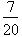of the 240 people are swimmers.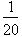of 240 = 12, soof 240 = 7 x 12 = 84